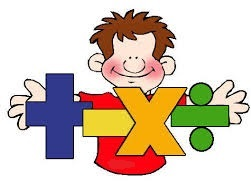Problems
205 - Mr. Ant’s Confusing Sum

Mr. Ant’s Confusing Sum

Time Limit: 1 sec

The Problem

Mr. Ant add two numbers in an interesting way. He adds two numbers such as

1 + 2 = 2

2 + 2 = 4

3 + 3 = 9

4 + 4 = 16

One day Mr. Ant’s friend Miss. Butterfly came for a visit at Mr. Ant’s home. Mr. Ant asked Miss Butterfly can you add two numbers. She became confuse, can you help Miss. Butterfly with this?The Input

The input file contains 2 integer numbers A and B (A<300 and B<300).

The Output

Output will show the confusing sum of A and B in a separate line.

Sample Input

25 30

Sample Output

750

Problem Setter: Samia Safa Ahmed